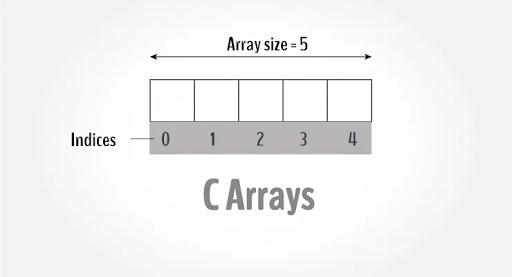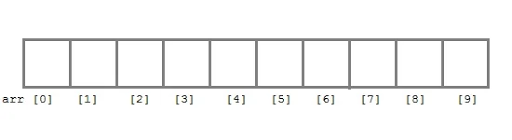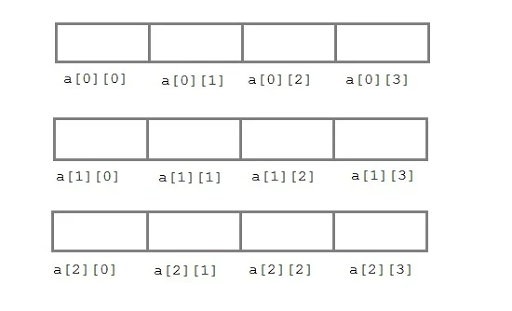## Pages

### C Programming: Arrays in 'C' LanguageIn C language, `arrays` are referred to as structured data types. An array is defined as a finite ordered collection of homogenous data, stored in contiguous memory locations.

Here the words,

• finite means data range must be defined.
• ordered means data must be stored in contiguous memory addresses.
• homogenous means data must be of a similar data type.

### An example where arrays are used,

• to store the list of Employee or Student names,
• to store marks of students,
• or to store the list of numbers or characters, etc.

Since arrays provide an easy way to represent data, it is classified amongst the data structures in C. Other data structures in C are structurelistsqueuestrees, etc. The array can be used to represent not only a simple list of data but also the table of data in two or three dimensions.

### Declaring an Array

Like any other variable, arrays must be declared before they are used. The general form of array declaration is,

``````data-type variable-name[size];

/* Example of array declaration */

int arr;``````Here`int`is the data type, `arr` is the name of the array and 10 is the size of the array. It means the array`arr`can only contain 10 elements of`int`types.

Index of an array starts from `0` to size-1 i.e first element of `arr` the array will be stored at `arr` address and the last element will occupy `arr`.

### Initialization of an Array

After an array is declared it must be initialized. Otherwise, it will contain a garbage value(any random value). An array can be initialized at either compile-time or at runtime.

#### Compile-time Array initialization

Compile-time initialization of array elements is the same as ordinary variable initialization. The general form of initialization of array is,

``````data-type array-name[size] = { list of values };

/* Here are a few examples */
int marks={ 67, 87, 56, 77 };    // integer array initialization

float area={ 23.4, 6.8, 5.5 };   // float array initialization

int marks={ 67, 87, 56, 77, 59 };    // Compile time error``````

One important thing to remember is that when you will give more initializers (array elements) than the declared array size then the compiler will give an error.

``````#include<stdio.h>

void main()
{
int i;
int arr[] = {2, 3, 4};      // Compile time array initialization
for(i = 0 ; i < 3 ; i++)
{
printf("%d\t",arr[i]);
}
}``````

OUTPUT: 2 3 4

#### Runtime Array initialization

An array can also be initialized at runtime using`scanf()`the function. This approach is usually used for initializing large arrays or to initialize arrays with user-specified values. Example,

``````#include<stdio.h>

void main()
{
int arr;
int i, j;
printf("Enter array element");
for(i = 0; i < 4; i++)
{
scanf("%d", &arr[i]);    //Run time array initialization
}
for(j = 0; j < 4; j++)
{
printf("%d\n", arr[j]);
}
}``````

### Two-dimensional Arrays

C language supports multidimensional arrays also. The simplest form of a multidimensional array is the two-dimensional array. Both the row's and column's index begins from `0`.

Two-dimensional arrays are declared as follows,

``````data-type array-name[row-size][column-size]

/* Example */
int a;``````An array can also be declared and initialized together. For example,

``````int arr[] = {
{0,0,0},
{1,1,1}
};``````

Note: We have not assigned any row value to our array in the above example. It means we can initialize any number of rows. But, we must always specify the number of columns, else it will give a compile-time error. Here, a `2*3` multi-dimensional matrix is created.

#### Runtime initialization of a two dimensional Array

``````#include<stdio.h>

void main()
{
int arr;
int i, j, k;
printf("Enter array element");
for(i = 0; i < 3;i++)
{
for(j = 0; j < 4; j++)
{
scanf("%d", &arr[i][j]);
}
}
for(i = 0; i < 3; i++)
{
for(j = 0; j < 4; j++)
{
printf("%d", arr[i][j]);
}
}
}``````

#### KING YT

© All post,blogs,stories,etc. written/posted here is the property of KING YT